Beginners Guide to Markov Chains

Introduction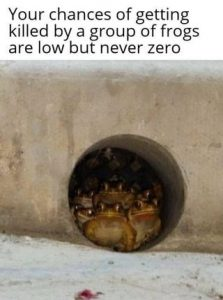Hello and welcome to the wonderful world of Probability where as math teachers say that the probability of many strange things that could happen is not equal to zero.

I’ll try to explain the topic in a simple way so that even those who are not so great mathematicians (including me) will understand.

Definition

Markov random processes or Markov chains are named for the outstanding Russian mathematician A.A. Markov (1856-1922), who first began the study of the probabilistic relationship of random variables and created a theory that can be called “dynamics of probabilities.” In the future, the foundations of this theory were the starting point of the general theory of random processes, and many important applied sciences, such as reliability theory, queuing theory, etc. Nowadays the theory of Markov processes is widely used in various fields of sciences such as mechanics, physics, chemistry, etc.

Due to the comparative simplicity and visibility of the mathematical apparatus, the high reliability and accuracy of the decisions obtained, Markov processes gained particular attention from specialists involved in operations research and the theory of optimal decision making.

Markov processes is a mathematical system that jumps (passes) from one state to another randomly. System status is user defined.

These may be situations or quantities (parameters). I will show you several states related to passing the OCA exam that could be used with Markov chains as an example.

Markov processes describe the behavior of systems whose states depend only on the previous state. This dependence is specified using the probability of transitions from one state to another (conditional probability).If I read the OCA exam guides I will most likely pass the exam successfully, but there is always a chance that I will fail (maybe my stomach will growl due to the fact that I ate too little in the morning, and this won’t let me concentrate).

If I read comic books I will most likely fail the exam then, but there is still a chance that I can pass the exam.

Directed graph

It is convenient to graphically describe Markov processes using directed graphs. A directed graph is a set of vertices and arrows connecting these vertices.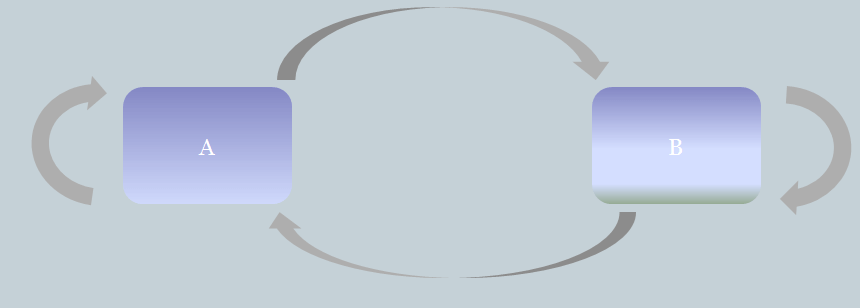If you see that the arrows are marked with numbers (quantities) – this is a weighted graph.

Weighted graph

Weighted directed graphs are used to represent Markov processes.The figure above shows an oriented graph of a patient’s transitional states. In this case, the patient can move from the state of “Healthy” to “Sick”, and vice versa, and also there is a probability to remain in the same state in which he is already. The numbers above the arrows are the approximate probabilities of transition from one state to another (the figure shows that the probability of transition from “Healthy” to “Sick” is 0.3; the probability of staying in the same state is 0.7).

It should be taken into consideration that the sum of all the probabilities emanating from one of the states in the sum should give 1.

An example of a more complex oriented graph is the graph of child behavior that I created. I took 4 states in which a child can be (plays, sleeps, eats, cries), and noted the approximate probabilities of transitions from one state to another. (I cannot vouch for the accuracy of probabilities, since I have no children yet).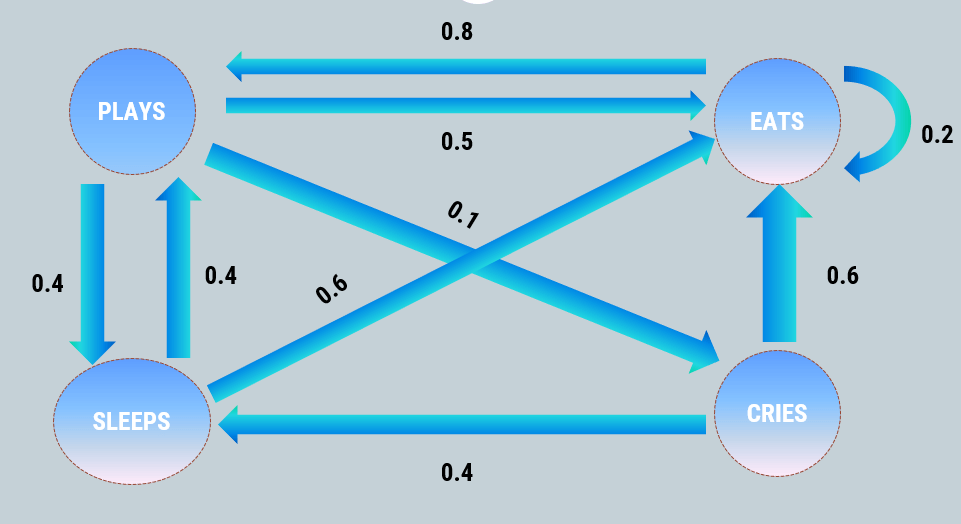Application and necessary formulas on real life examples

I won’t go too much into details in terms of formulas and calculations, since a full description of all the things could lead to a new scientific research article and maybe help me to get a doctorate degree. I believe that everything new is easier to learn from examples, so let’s move on to one.

Let’s consider that the management of the company “Guguta & Co Inc.” divides employees into two groups: prone to disease, and resistant.

An employee fits into the group “prone to diseases” if he has been ill at least once in the past 12 months, and in the group “resistant to diseases” if he has never been ill during the past 12 months.

Based on statistics, the company found that if a person is “prone to diseases”, the risk of remaining in the same group is 70%, and the chance to move to the group of “resistant to diseases” is 30%.

At the same time, if a person is in the “disease-resistant” group, the chance to move to the “disease-prone” group is 20%, and the chance to stay in the same group is 80%.

Using Markov processes, the director can calculate approximately what state the employee will be in after some time (in our case, after several years), knowing his initial state.

First, we will depict the probabilities of transition from one state to another using the figures and construct a weighted graph then.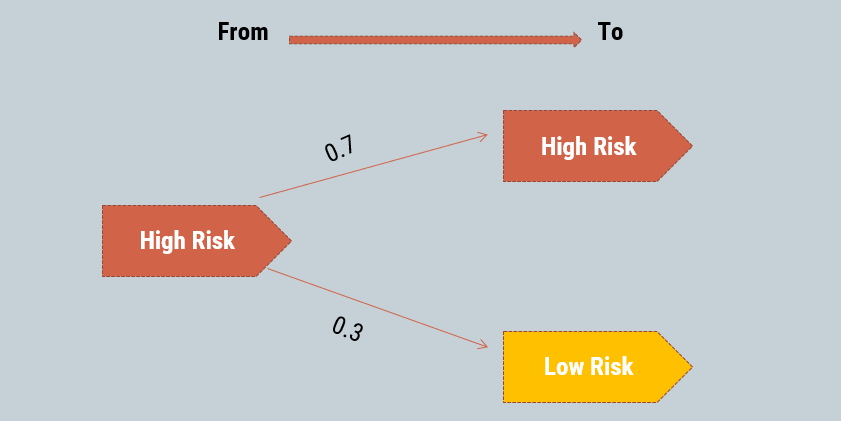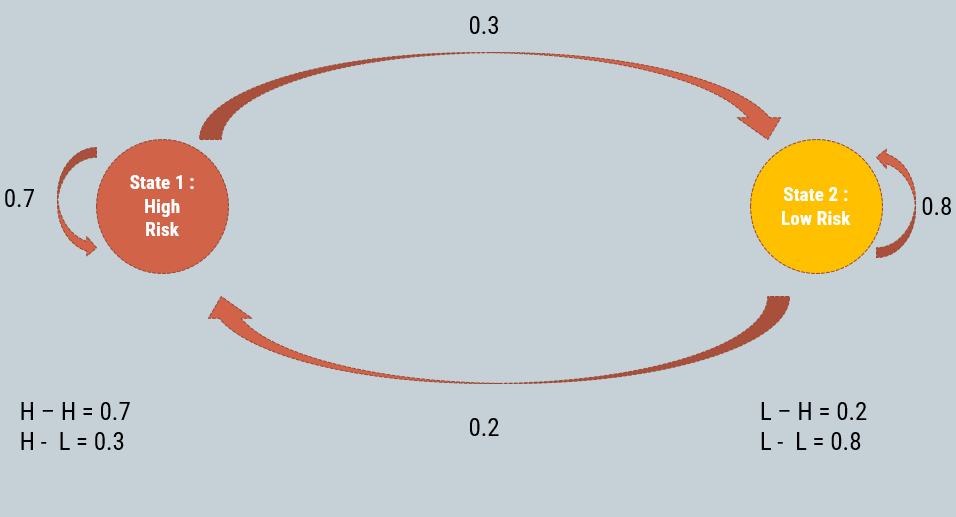H stands for High Risk, L stands for Low Risk.

It is customary to compose a matrix of transition states to calculate the probability. Its construction can be seen in the picture below. We write out the same data as in the weighted graph, but in the form of a matrix.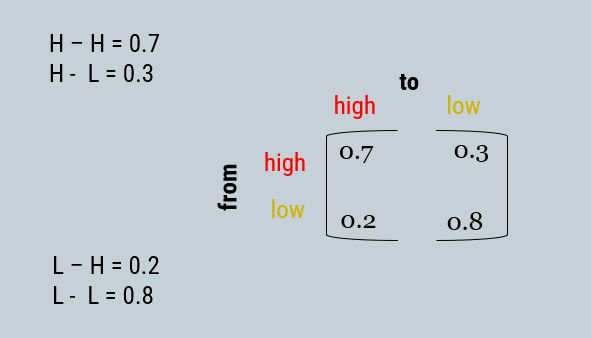Calculating the probability

To calculate the probability we need an initial state vector. It sets the initial state of the system, or the probability of a transition from one state to another.

In my example, the firm calculated that a randomly selected employee has a probability of moving to the “high risk” group is 0.1, and the probability of moving to a low risk group is 0.9.To calculate the probability after one step (in our case, one year), it is necessary to multiply the vector of the initial state by the matrix of transition states.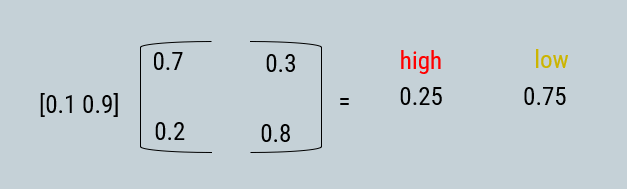As a result, it turns out that the employee has a probability of going into the “low growth” group is 0.75, and the probability of going into the “high risk” group is 0.25.

The formula for calculating the probability is:

v * P * P * P … * P (m times) = v * P ^ m

where m is the number of steps that we want to calculate, v is the vector of the initial state, and P is the matrix of transition states. If we want to calculate the probability after 2 years, we need to raise the matrix of transition states to the second power then. Examples of calculating probabilities after 2 years, 4 years, and 6 years are presented in the picture below.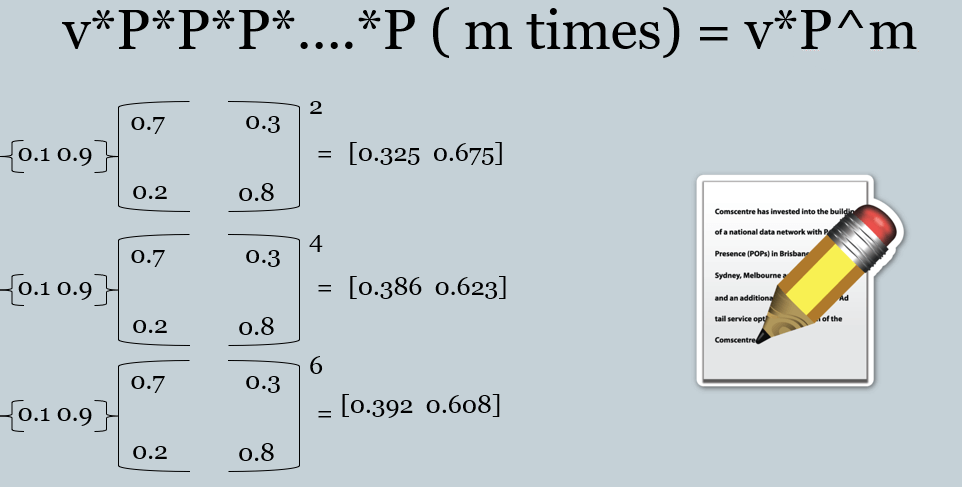Using Markov’s processes in game development

Markov processes can be used also in game development to increase a variety of behavior of game characters.

In this example I have created an orc behavior model, drew a weighted graph, and calculated the approximate state of the orc after 2 steps.

In my example an orc can be in five states: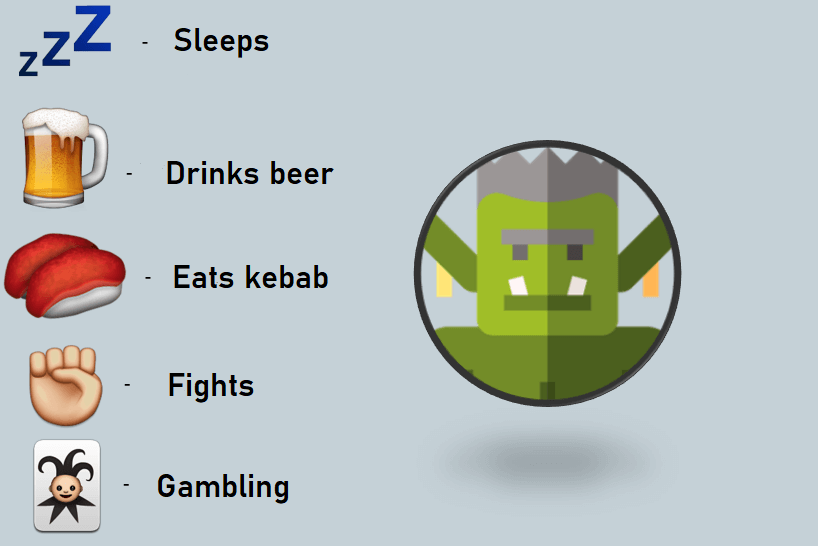A scheme with calculated probabilities looks like that:Unfortunately I wasn’t able to observe the behaviour of a real life orc, so the numbers are probably inaccurate.

Such a large scheme does not bring much geometric joy, so we will construct a matrix of transition states.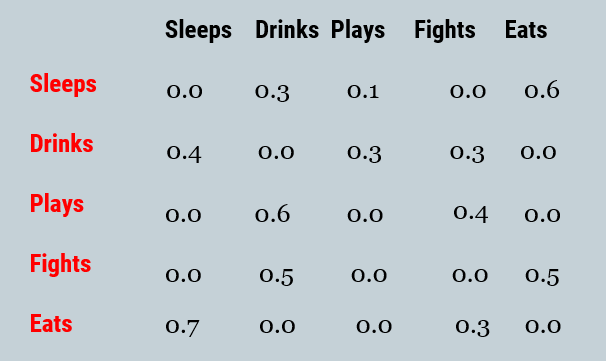Then we calculate the probability after 3 steps. In our case, the initial state vector is [0.0 1.0 0.0 0.0 0.0] (Orc is initially in the “drinking” state). We multiply the initial state vector by the transition state matrix to the power of 2 and obtain approximate probabilities.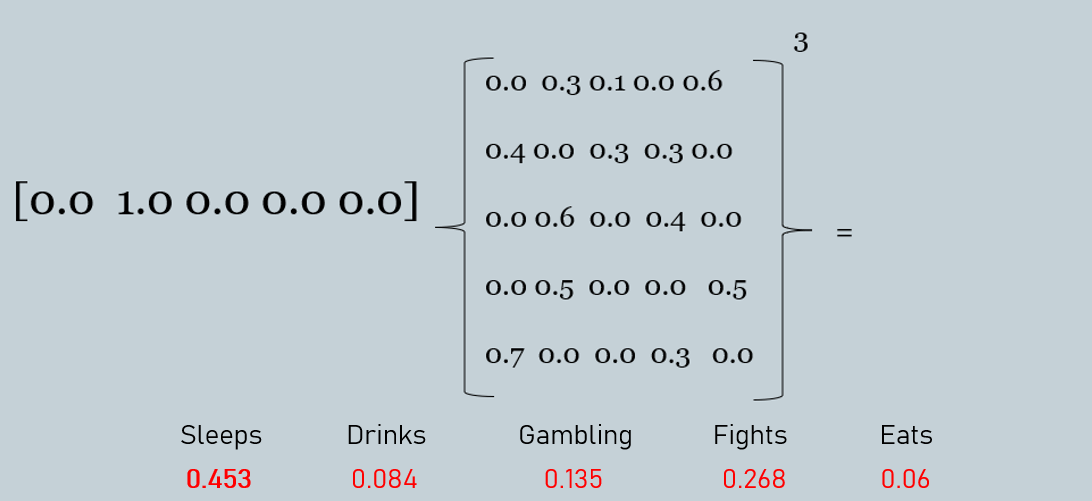As a result it turns out that being on start in the “drinking” state, after 3 steps the orc will most likely be in the “sleeping” state (This result is very “unexpected”, wasn’t it? 😊).

Weather forecasting

With help of Markov processes and statistics you can also predict the weather. My guess is that Yandex.Weather most likely uses Markov processes as one of the parts of core algorithms in combination with neural networks.

I made a model of transitional weather conditions as an example. In this example, the weather can be in one of three states: “clear”, “cloudy” and “slightly cloudy”.

First thing we must do is to construct a weighted graph.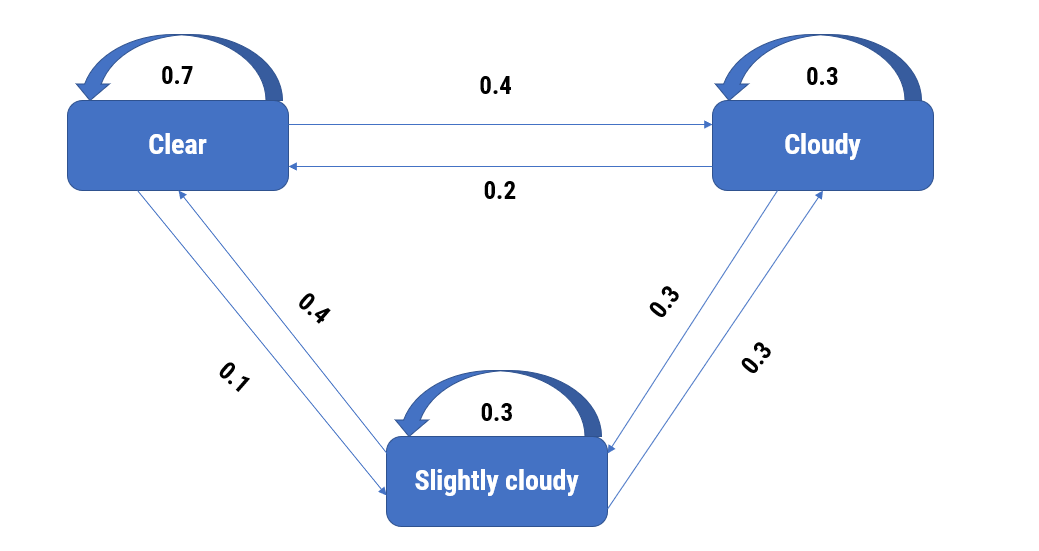Next, we will calculate the approximate weather condition after 2 steps, if the weather was clear initially.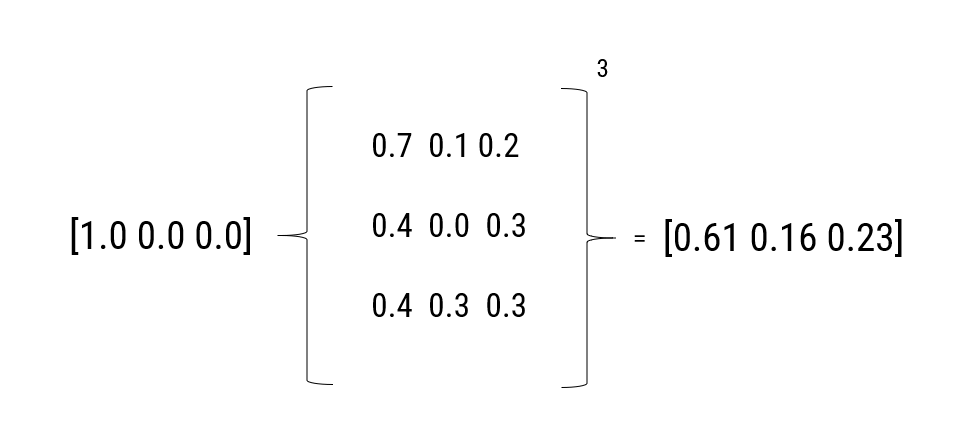Conclusion

I consider that the use of Markov processes is very promising. The scope is quite wide, Markov processes can be used in many domains such as: economics, sports, meteorology, and computer games. It is a very useful tool for predicting future events. For example, you can predict whether customers will move from one product to another, sales forecasting, weather forecasting, predict the condition of patients, and even help the football team captain to consider which player will most likely score a decisive goal. In addition, the calculation of the predictions of system states uses algorithms which could be easily implemented in practice using programming languages.

I hope you enjoyed this article and didn’t fall asleep during the reading process. I tried to make a simplified explanation of a quite difficult topic, and there are many more things left that should be described too. This article was intended by me as a short introduction for those who are interested in probability theory and possibly get the attention of new fans.

See you all in the next article! (or in the office / Skype)

So Long, and Thanks for All the Fish.